# Electronics - RLC Circuits and Resonance

### Exercise :: RLC Circuits and Resonance - True or False

1.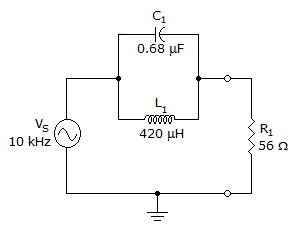With the frequency given, the circuit in the given circuit is operating above resonance.

 A. True B. False

Answer: Option A

Explanation:

No answer description available for this question. Let us discuss.

2.

The current through the inductor is 5 amps.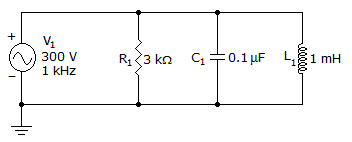A. True B. False

Answer: Option B

Explanation:

No answer description available for this question. Let us discuss.

3.

Resonance will occur when XC = XL in the circuit below.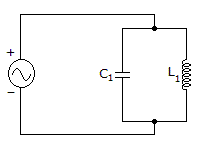A. True B. False

Answer: Option A

Explanation:

No answer description available for this question. Let us discuss.

4.

The resonant frequency fo is 19 kHz.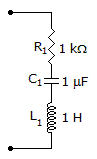A. True B. False

Answer: Option B

Explanation:

No answer description available for this question. Let us discuss.

5.

The impedance is 1177.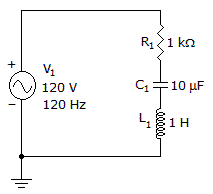A. True B. False

Answer: Option A

Explanation:

No answer description available for this question. Let us discuss.

#### Current Affairs 2021

Interview Questions and Answers